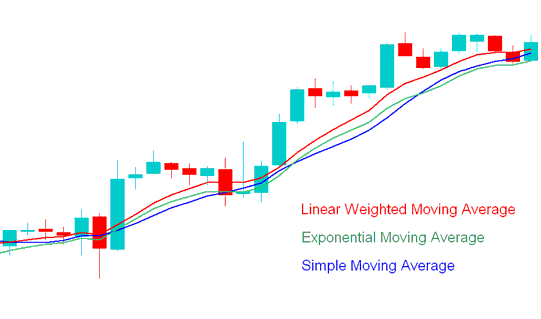# Types of Indices Moving Averages

There are four types of indices trading moving averages:

1. Simple indices trading moving average

2. Exponential indices trading moving average

3. Smoothed indices trading moving average

4. Linear weighted indices trading moving average

The difference between these 4 indices trading moving averages is the weight assigned in to the most recent stock indexes price data.

## Simple Moving Average

Indices SMA indicator applies equal weight to the indices trading data used to calculate the simple moving average and is calculated by summing up the stock indexes price periods of a stock indices chart and this value is then divided by the number of such stock indexes price periods. For example indices trading simple moving average 10, adds the stock indexes price data for the last 10 stock indexes price periods and divides them by 10.

## Exponential Moving Average

Indices EMA indicator applies more weight to the most recent stock indexes price data and is calculated by assigning the latest stock indexes price values more weight based on a percent P, multiplier that is used to multiply and assign more weight to the latest stock indexes price data.

## Linear Weighted Moving Average

Indices LWMA indicator moving averages applies more weight to the most recent stock indexes price data and the latest data is of more value than earlier stock indexes price data. Linear Weighted indices trading moving average is calculated by multiplying each of the indices trading closing indices prices within the series, by a certain weight coefficient.

## Smoothed Moving Average

Indices SMMA Indicator is calculated by applying a smoothing factor of N, the smoothing factor is composed of N smoothing for N stock indexes price periods.

The stock indices chart example explained and illustrated below shows SMA, EMA and LWMA. The SMMA indices trading moving average is not commonly used so it is not shown below.

The LWMA stock indices indicator reacts fastest to stock indexes price data, followed by the EMA and then the SMA.SMA, LWMA, EMA - Types of Indices Moving Averages - SMA, EMA and LWMA# Day Trading Indices with Exponential and Simple Moving Averages

The SMA and EMA indices trading moving averages are the most commonly used Moving averages to trade indices. Whereas the EMA indices trading moving average has a more sophisticated method of calculation, its more popular than the SMA indices trading moving average.

Simple Moving Average is the arithmetic mean of the closing indices prices in the stock indexes price period based on the set time period where each time period is added and then it is divided by the number of time stock indexes price periods chosen. If 10 is the stock indexes price period used the stock indexes price for the last ten stock indexes price periods added up then it is divided by 10.

SMA stock indices indicator is the result of a simple arithmetic average. Very simple and some Indices traders tend to associate with the indices trend since it closely follows stock indexes price action.

EMA on the other hand uses an acceleration factor and it is more responsive to the indices trend.

The SMA indices trading moving average is used in stock indices charts to analyze stock indexes price action. If the stock indexes price action in more than 3 or 4 time stock indexes price periods the SMA then it's an indication that long stock indexes trades should be closed immediately and the bullish momentum of the buy indices trade is waning.

The shorter the SMA stock indexes price period the faster it is to respond to stock indexes price change. SMA stock indices indicator can be used to show direct information regarding the indices trend of the stock indexes trading market and the strength by looking at its slope, the steeper or more pronounced slope of the SMA is, the stronger the Indices trend.

The Exponential Moving Average is also used by many indices traders in the same way but it reacts faster to the stock indexes trading market moves and therefore it is more preferred by some stock indexes traders.

The SMA and EMA can also be used to generate entry and exit points when indices trading. These Moving averages can also be combined with Fibonacci and ADX indices indicators to generate confirmation the stock indices signals generated by these moving averages.